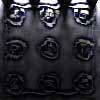#### You may also like### Coin Tossing Games

You and I play a game involving successive throws of a fair coin. Suppose I pick HH and you pick TH. The coin is thrown repeatedly until we see either two heads in a row (I win) or a tail followed by a head (you win). What is the probability that you win?### Scratch Cards

To win on a scratch card you have to uncover three numbers that add up to more than fifteen. What is the probability of winning a prize?### Factoring a Million

In how many ways can the number 1 000 000 be expressed as the product of three positive integers?

# Flora the Florist

##### Age 14 to 16 ShortChallenge Level

Using the highest common factor
The number of bunches must divide the number of white roses, the number of yellow roses and the number of red roses.

The highest common factor of $24$, $36$ and $42$ is $6$, so the most bunches Flora can make is $6$, each consisting of $4$ white, $6$ yellow and $7$ red roses.

Using ratio
Use all the roses to make identical bunches - so all bunches will have the same ratio of colours as the total
$24:42:36$
$12:21:18$
$4:7:6$
Cannot simplify further so each bunch has $4$ white roses
So Flora can make $6$ from $24$ white roses

This problem is taken from the UKMT Mathematical Challenges.
You can find more short problems, arranged by curriculum topic, in our short problems collection.